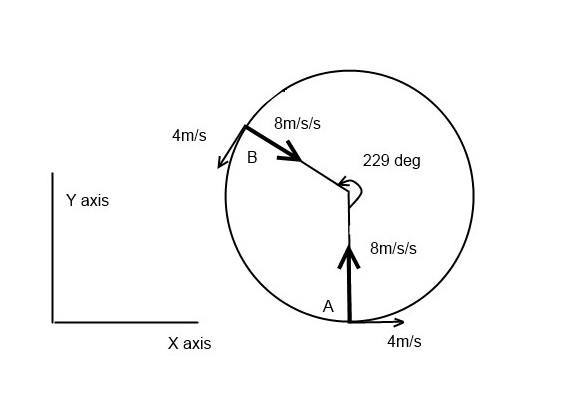# Finding angle of velocity

• Nousher Ahmed
In summary, the conversation discusses two mathematical problems related to centripetal force and uniform circular motion. The first problem involves determining the direction of velocity if force acts at a right angle, while the second problem involves finding the angle between the initial and final velocity. The conversation also addresses misunderstandings about the effect of acceleration on velocity and emphasizes the importance of considering velocity as a vector with both speed and direction components.

#### Nousher Ahmed

This is not homework. This problem has been made by me. One day ago, I asked help about how to determine the direction of velocity if force act with velocity at right angle. After asking help, some comments helped me to give some better idea. Then I made this problem with some values to realize that fact easily. But I found some problems for which I want to post this mathematical problem at this site. If it is necessary, please edit this post as necessary.

Here we will try to solve two mathematical problems related to centripetal force. This two problems are interrelated to each other. Suppose, a particle is traveling through a circular path with uniform value of velocity. Assume that, value of velocity, v= 4 m/s, mass of particle, m= 2 kg, radius of circle, r= 2 m. Hence by calculating we get the value of centripetal force, F= 16 N, acceleration, a= 8 m/s², angular frequency, w= 2 rad/second.

Let, at t=0, particle is at point A of circle. and, at t=2, particle is at point B of circle. The angle produced by arc AB at the centre of circle is= w*t =4 rad= 229.2 degree.

Now we will determine the angle between the angle of direction of initial velocity of particle (when t=0) and the direction of final velocity (at t=2). This angle equals to the angle produced by arc AB at the centre of circle. Geometrically it can be proved easily that these two angles are equal. Let, at t=0, the direction of velocity of particle is parallel to the direction of X axis. As a result, along X axis, the value of initial velocity is 4 m/s, and along Y axis, it is 0. Since the centripetal force acts at right angle with the direction of initial velocity, along X axis the value of velocity will not change. It will change along X axis only. After 2 seconds, the value of velocity along Y axis will be 16 m/s. And at this moment the direction of resultant velocity with X axis can be determined by the following way. tan∅= (16/4)= 4, ∅= 75.96 degree. This angle is not equal to the angle produced by arc AB at the centre of circle. Please help me to find out the error. If it is possible to you, please show the correct way to solve it.

Nousher Ahmed said:
As a result, along X axis, the value of initial velocity is 4 m/s, and along Y axis, it is 0.
Nousher Ahmed said:
After 2 seconds, the value of velocity along Y axis will be 16 m/s.
You're contradicting yourself. You first mentioned that the velocity of the particle which is in circular motion does not change. How can you then state that the velocity at t=2 is 16m/s ? I guess you've misunderstood the effect of acceleration here - it does not change the magnitude of the velocity, but rather changes the direction. The magnitude of the velocity at "Y" will be 4 m/s as well.

I suspect PWiz is correct. eg You misunderstand the effect of acceleration.

As a result, along X axis, the value of initial velocity is 4 m/s, and along Y axis, it is 0.

Ok so point A is at a position similar to that shown in my drawing (eg where X is changing but Y isn't).Since the centripetal force acts at right angle with the direction of initial velocity, along X axis the value of velocity will not change. It will change along X axis only. After 2 seconds, the value of velocity along Y axis will be 16 m/s.

No. That would only happen if you stopped the object at point A and applied a force that accelerated it vertically. That's not what's happening. The object is constrained to move in a circle. At point A the X component of the velocity might not be changing but it isn't zero.

I think you need to remember velocity is a vector that has components speed and direction. In the case of uniform circular motion the speed is constant but the direction is changing. We only really say it's "accelerating towards the centre" because ANY change of velocity is an acceleration. An acceleration does not imply a change in speed, it can (and does in this case) mean a change in the direction component of velocity.